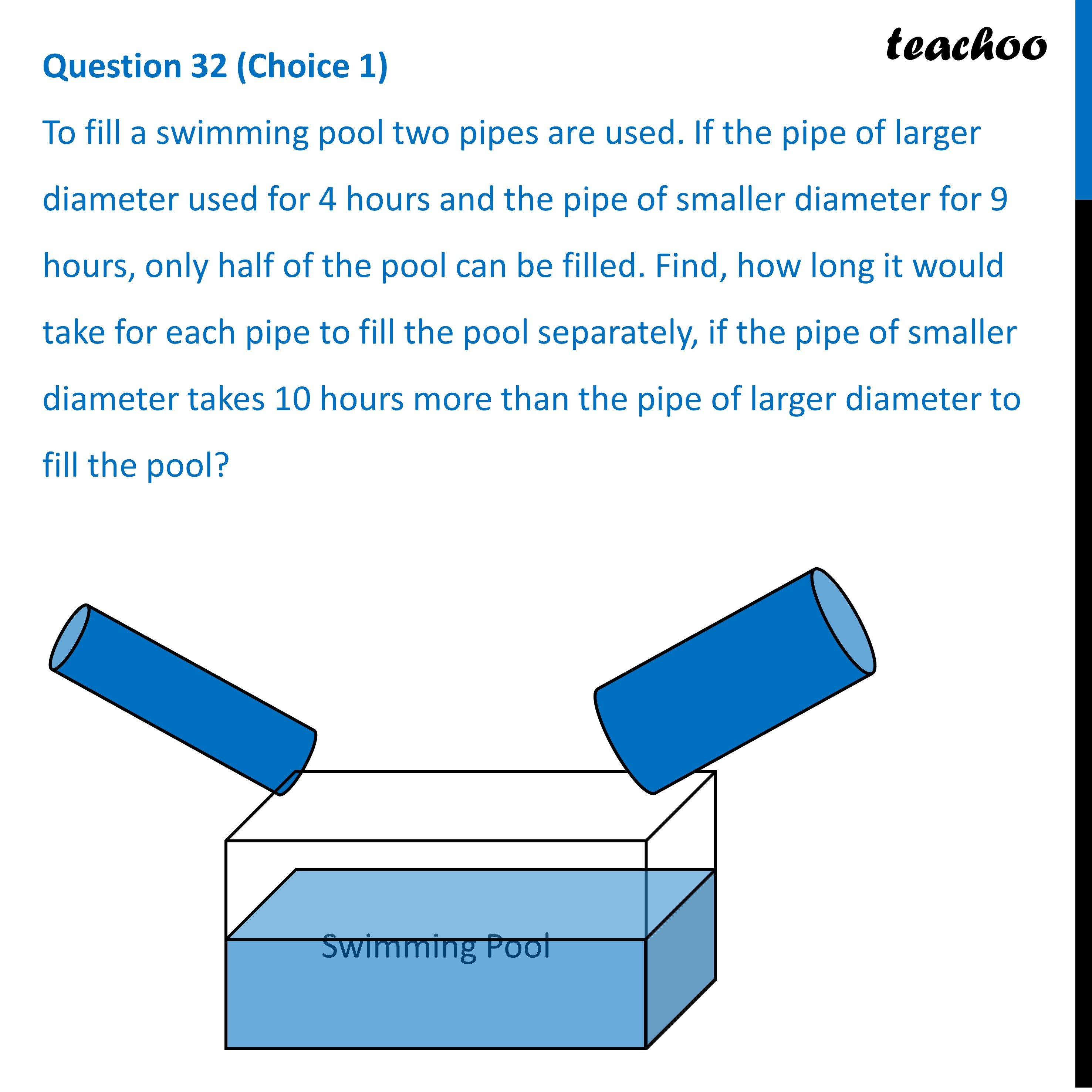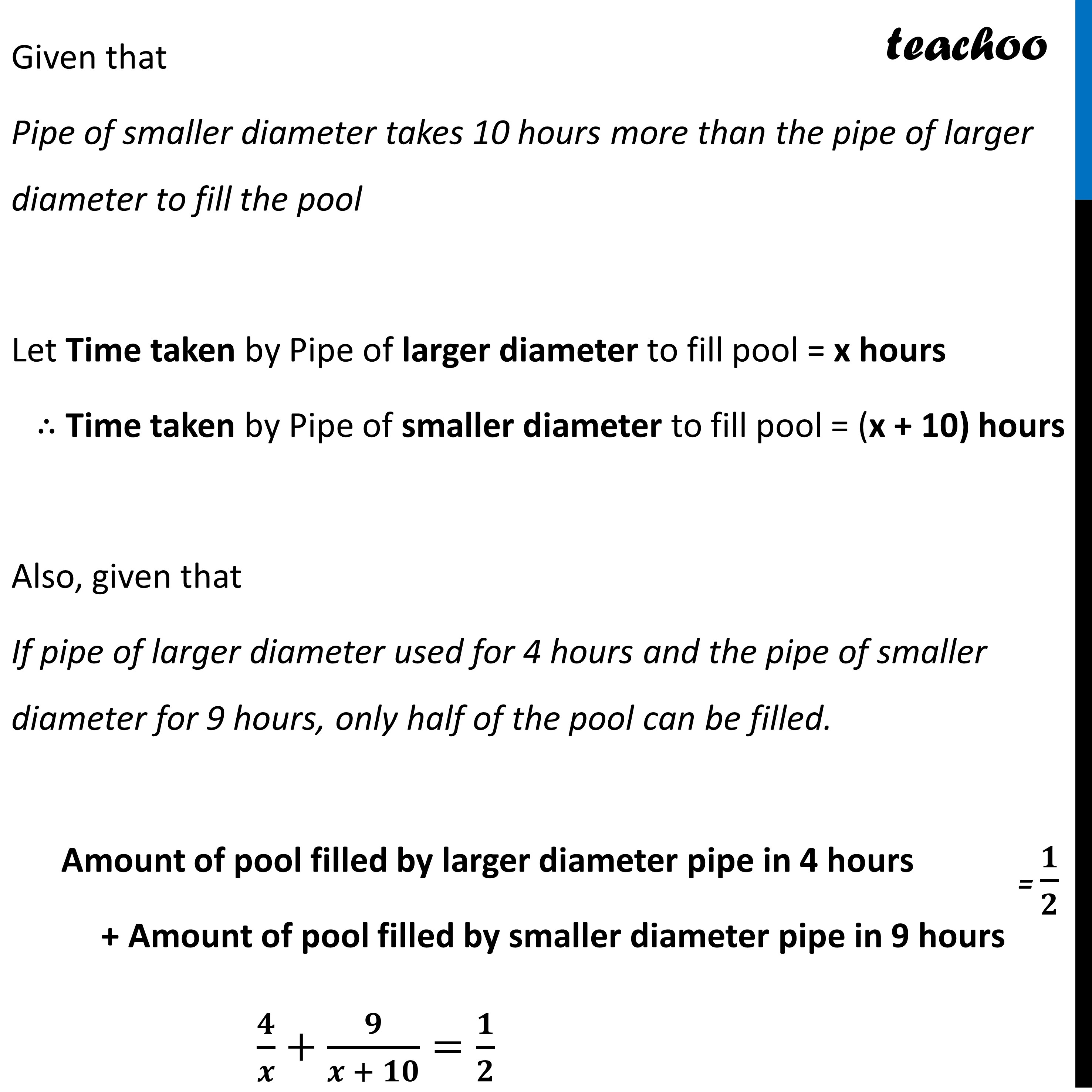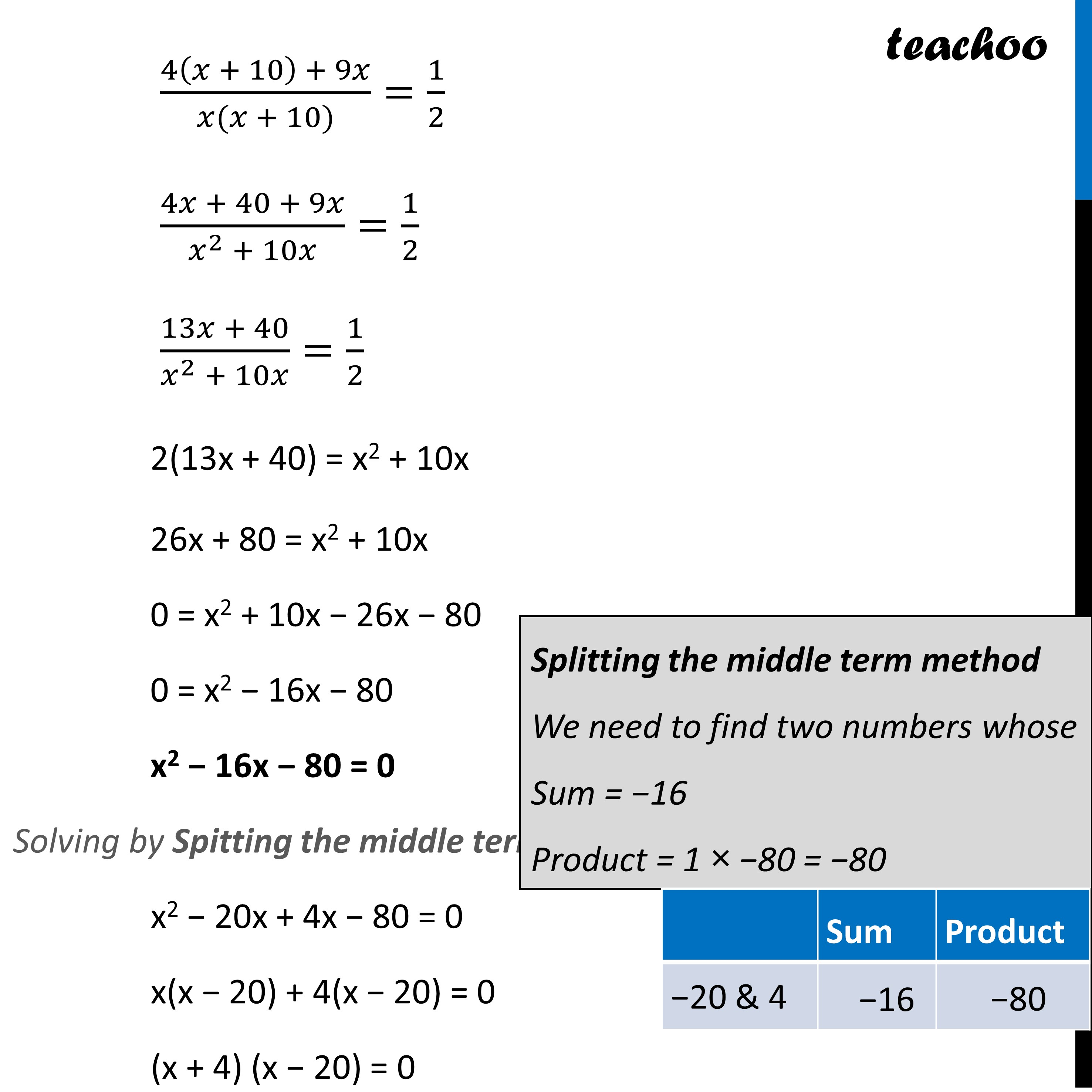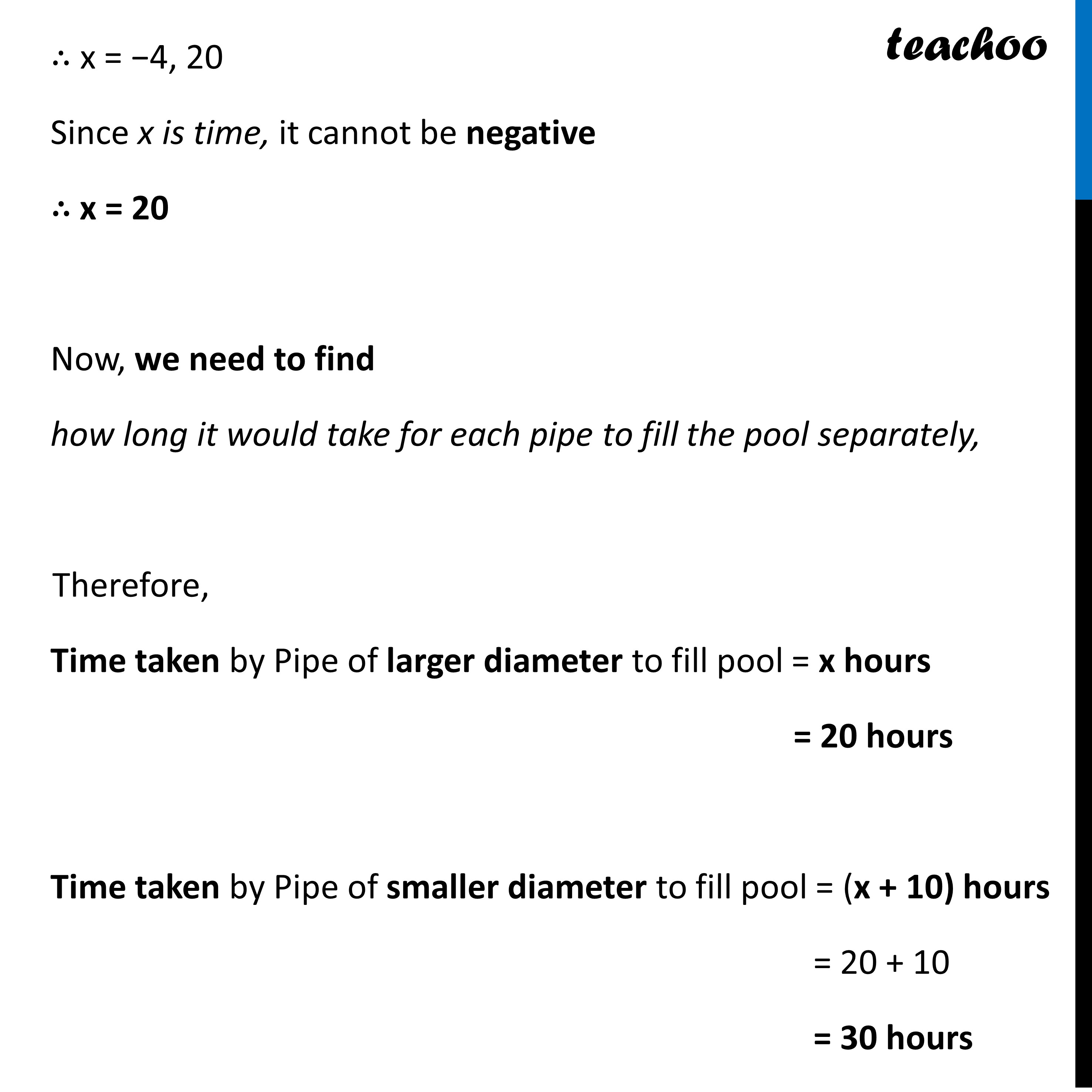CBSE Class 10 Sample Paper for 2023 Boards - Maths Standard

Class 10
Solutions of Sample Papers for Class 10 Boards

## To fill a swimming pool two pipes are used. If the pipe of larger diameter used for 4 hours and the pipe of smaller diameter for 9 hours, only half of the pool can be filled. Find, how long it would take for each pipe to fill the pool separately, if the pipe of smaller diameter takes 10 hours more than the pipe of larger diameter to fill the pool?Learn in your speed, with individual attention - Teachoo Maths 1-on-1 Class

### Transcript

Question 32 (Choice 1) To fill a swimming pool two pipes are used. If the pipe of larger diameter used for 4 hours and the pipe of smaller diameter for 9 hours, only half of the pool can be filled. Find, how long it would take for each pipe to fill the pool separately, if the pipe of smaller diameter takes 10 hours more than the pipe of larger diameter to fill the pool?Given that Pipe of smaller diameter takes 10 hours more than the pipe of larger diameter to fill the pool Let Time taken by Pipe of larger diameter to fill pool = x hours ∴ Time taken by Pipe of smaller diameter to fill pool = (x + 10) hours Also, given that If pipe of larger diameter used for 4 hours and the pipe of smaller diameter for 9 hours, only half of the pool can be filled. Amount of pool filled by larger diameter pipe in 4 hours + Amount of pool filled by smaller diameter pipe in 9 hours 𝟒/𝒙+𝟗/(𝒙 + 𝟏𝟎)=𝟏/𝟐 (4(𝑥 + 10) + 9𝑥)/(𝑥(𝑥 + 10))=1/2 (4𝑥 + 40 + 9𝑥)/(𝑥^2 + 10𝑥)=1/2 (13𝑥 + 40)/(𝑥^2 + 10𝑥)=1/2 2(13x + 40) = x2 + 10x 26x + 80 = x2 + 10x 0 = x2 + 10x − 26x − 80 0 = x2 − 16x − 80 x2 − 16x − 80 = 0 Solving by Spitting the middle term x2 − 20x + 4x − 80 = 0 x(x − 20) + 4(x − 20) = 0 (x + 4) (x − 20) = 0 Splitting the middle term method We need to find two numbers whose Sum = −16 Product = 1 × −80 = −80 ∴ x = −4, 20 Since x is time, it cannot be negative ∴ x = 20 Now, we need to find how long it would take for each pipe to fill the pool separately, Therefore, Time taken by Pipe of larger diameter to fill pool = x hours = 20 hours Time taken by Pipe of smaller diameter to fill pool = (x + 10) hours = 20 + 10 = 30 hours